# Partnership - Quantitative Aptitude (MCQ) questions

Dear Readers, Welcome to Quantitative Aptitude Partnership questions and answers with explanation. These Partnership solved examples with shortcuts and tricks will help you learn and practice for your Placement Test and competitive exams like Bank PO, IBPS PO, SBI PO, RRB PO, RBI Assistant, LIC,SSC, MBA - MAT, XAT, CAT, NMAT, UPSC, NET etc.

After practicing these tricky Partnership multiple choice questions, you will be exam ready to deal with any objective type questions.

1)   P gave Rs. 12000 for starting a business. Q gave Rs. 16000. What will be share of profit of R after 2 years, if R comes after 8 months with his Rs. 15000 and profit earned by P, Q and R is Rs. 45600?
- Published on 03 May 17

a. Rs. 24000
b. Rs. 12000
c. Rs. 6000
d. Rs. 18000
 Answer  Explanation ANSWER: Rs. 12000 Explanation: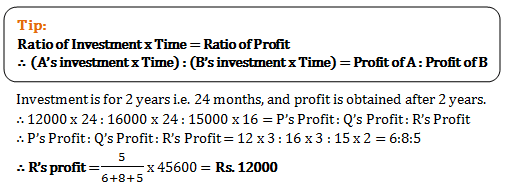2)   Rs. 15000 were invested by Ram and Shyam together to start a small business. They got a profit of Rs. 2000 at the end of the year. Shyam took his profit share of Rs. 600. How much did Ram invest?
- Published on 03 May 17

a. Rs. 9000
b. Rs. 4500
c. Rs. 10500
d. Rs. 11000
 Answer  Explanation ANSWER: Rs. 10500 Explanation: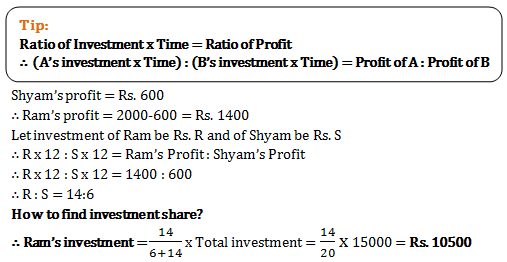3)   Jay, Veeru and Basanti start a travel agency. Jay contributes Rs. 9100, while Veeru and Basanti invest Rs. 6825 and Rs. 8190 respectively. If Jay, Veeru and Basanti invest for 3 months, 2 months and 5 months respectively. How much will Veeru get out of total profit of Rs. 4158?
- Published on 03 May 17

a. Rs. 693
b. Rs. 1036
c. Rs. 986
d. Rs. 1247
 Answer  Explanation ANSWER: Rs. 693 Explanation: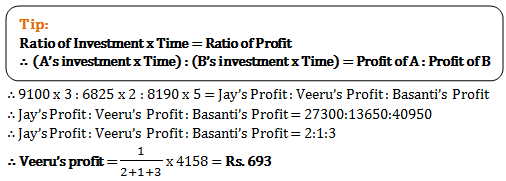4)   Neil started a business and Michael joined him after 2 months. Neil spent Rs. 30,000 while Michael spent Rs. 45,000. Their one year profit came out to Rs. 54000. How much profit will Neil get?
- Published on 03 May 17

a. Rs. 24000
b. Rs. 30000
c. Rs. 27000
d. Rs. 20000
 Answer  Explanation ANSWER: Rs. 24000 Explanation: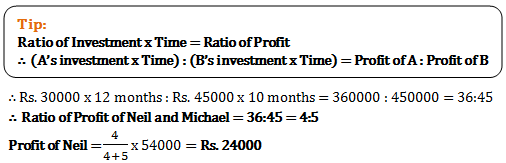5)   A small library is started by Prakash and John. Prakash invests Rs. 2000 and John Rs. 5000 for 9 and 7 months respectively. In what ratio will John and Prakash share the profit of Rs. 1100?
- Published on 03 May 17

a. 2:3
b. 21:27
c. 35:18
d. 45:56
 Answer  Explanation ANSWER: 35:18 Explanation: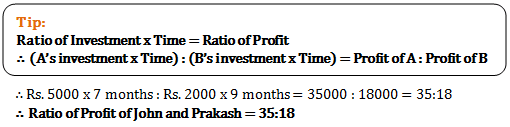6)   P and Q start a hotel. P left after 6 months. After the year ends, P gets Rs. 6000 as profit out of total profit of Rs. 9000. What will be amount invested by Q if P had invested Rs. 20000?
- Published on 13 Apr 17

a. Rs. 6000
b. Rs. 7500
c. Rs. 10000
d. Rs. 5000
 Answer  Explanation ANSWER: Rs. 5000 Explanation: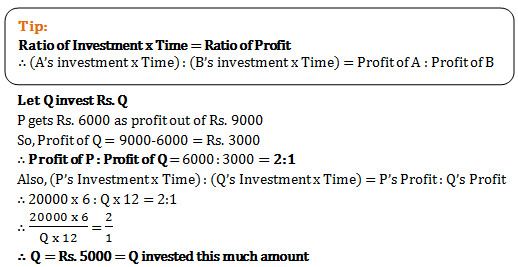7)   A started a travel agency using his Rs. 45000. B realized the profit in the business and joined after 3 months with his Rs. 60000. C came a bit late and after another 6 months invested Rs. 90000. Out of total profit of Rs. 20000 obtained after a year, what is share of C?
- Published on 12 Apr 17

a. Rs. 5000
b. Rs. 4000
c. Rs. 9000
d. Rs. 9500
 Answer  Explanation ANSWER: Rs. 4000 Explanation: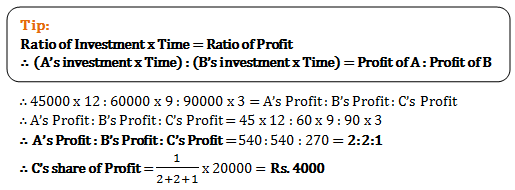8)   P and Q start a cafe with a capital Rs. 20,000 and Rs. 40,000 respectively. After a year, out of the profit of Rs. 15000, P gets his share of profit plus some money as his salary. In total if P gets Rs. 7000, what is the salary amount he received?
- Published on 12 Apr 17

a. Rs. 2000
b. Rs. 5000
c. Rs. 2500
d. Rs. 7500
 Answer  Explanation ANSWER: Rs. 2000 Explanation: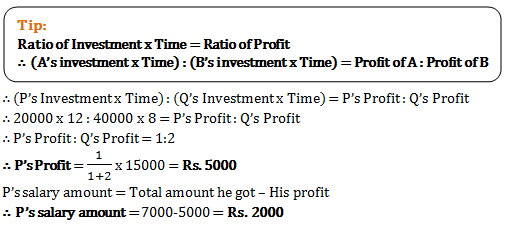9)   Ramesh and Suresh start a hotel. Ramesh invested Rs. 6000 and Suresh invested Rs. 5000. Ramesh’s investment was for 4 months and Suresh’s was for 8 months. If total profit was Rs. 6000, how much more profit than Ramesh did Suresh get?
- Published on 11 Apr 17

a. Rs. 1500
b. Rs. 4500
c. Rs. 3000
d. Rs. 1800
 Answer  Explanation ANSWER: Rs. 1500 Explanation: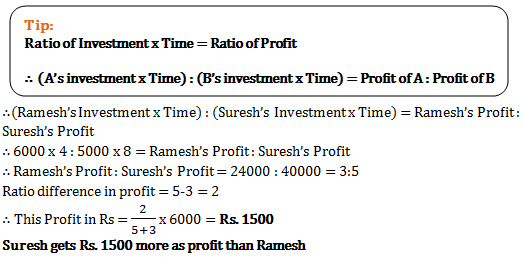10)   A, B, C and D invest in a business in the ratio 3:6:2:5 respectively. But the capital was utilized in the proportion 5:3:2:6 respectively. What is share of C out of a profit of Rs. 33500?
- Published on 11 Apr 17

a. Rs. 4000
b. Rs. 2000
c. Rs. 1000
d. Rs. 1500
 Answer  Explanation ANSWER: Rs. 2000 Explanation: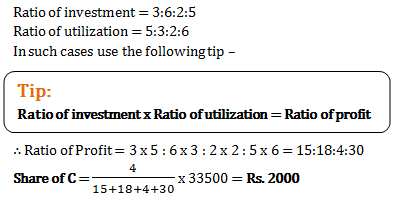1 2 3